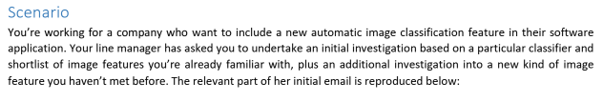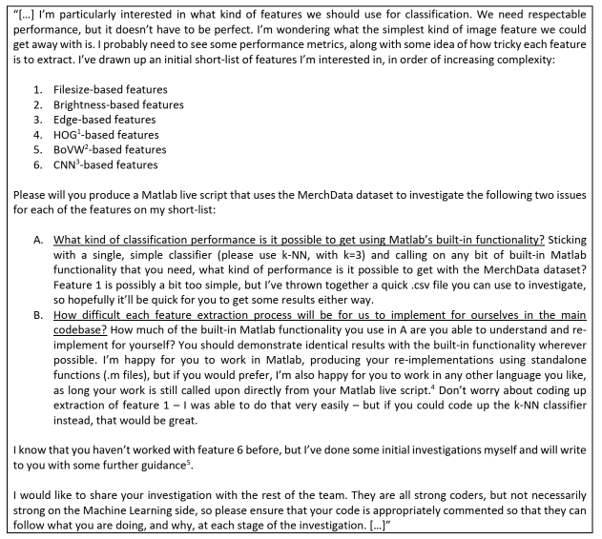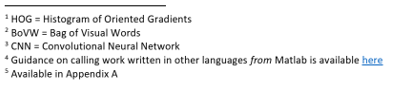# Create A Program to Create Image Classification in Matlab Assignment Solution.

## Instructions

Objective
Write a program to create image classification in matlab language.

## Requirements and SpecificationsSource Code

CALCULATE DISTANCE

% Description: calculate the Euclidean distance between any two % points % % Inputs: % p: an array containing the coordinates of the first point % q: an array containing the coordinates of the second point % % Outputs: % d: a numeric value holding the straight-line distance % between the two points % % Notes: you can find the equation for Euclidean distance in the % lecture slides. % function d = knn_calculate_distance(p, q)     % Guidance:     % 1. subtract the each of the feature values in p from the     % corresponding feature values in q     % 2. square each of the resulting differences     % 3. compute the total of those squared differences     % 4. compute the square root of that total value     % add your code and comments on the lines below:     % Step 1     d = q-p;     % Step 2     d = d.^2;     % Step 3     d = sum(d,2);     % Step 4     d = sqrt(d); end 

KNN FIT

% Description: create a model ready to perform k-NN classification % from some training data (assuming k=1 initially) % % Inputs: % train_examples: a numeric array containing the training examples % train_labels: a categorical array containing the associated % labels (i.e., with the same ordering as train_examples) % % Outputs: % m: a struct holding the parameters of the k-NN model (the % training examples, the training labels, and a value for k - the number of % nearest neighbours to use) % % Notes: % This method should be relatively short and simple, perhaps just a % few lines of code appropriately commented. % function m = knn_fit(train_examples, train_labels, k)     % Guidance:     % 1. store the supplied parameters as fields inside the model     % struct:     m = struct;     % add your code and comments on the lines below:     m.train_examples = train_examples;     m.train_labels = train_labels;     m.k = k; end 

KNN_PREDICT

% Description: use an existing k-NN model to classify some testing examples % % Inputs: % m: a struct containing details of the k-NN model we want to use % for classification % test_examples: a numeric array containing the testing examples we want to % classify % % Outputs: % predictions: a categorical array containing the predicted % labels (i.e., with the same ordering as test_examples) % % Notes: % Assumes that the model m has been created with a call to knn_fit() % function predictions = knn_predict(m, test_examples)     % Guidance (first task):     % 1. initialise an empty categorical array to hold the predictions     % 2. loop over every example in the testing_examples array     % and...     % a. find its nearest neighbour in the data inside the     % model     % b. take the label associated with that nearest neighbour as     % your prediction     % c. add the new prediction onto the end of your categorical     % array (from step 1)     % Guidance (second task):     % adjust your code to take account of the k value set inside the model     % m. Adjust step a from above so that all k nearest neighbours are     % found. Adjust step b from above to take the the most common class     % label across all k nearest neighbours as your prediction     % Step 1     predictions = categorical;     % Step 2     for i = 1:size(test_examples, 1)         p = test_examples(i,:); % current example         % Compute the distance of this example to all examples in the         % training dataset         d = knn_calculate_distance(m.train_examples, p);         % Sort the distances from lowest to highest         [d_sorted, idx] = sort(d);         % Pick k features/label for first train example since that's the nearest neighbor         nearest_x = m.train_examples(idx(1:m.k),:);         nearest_y = categorical(m.train_labels(idx(1:m.k)));         % Pick most commont feature         mostcommon_y = mode(nearest_y);         % Append prediction to the end of categorical array         predictions(i) = mostcommon_y;     end     % add your code and comments on the lines below: end